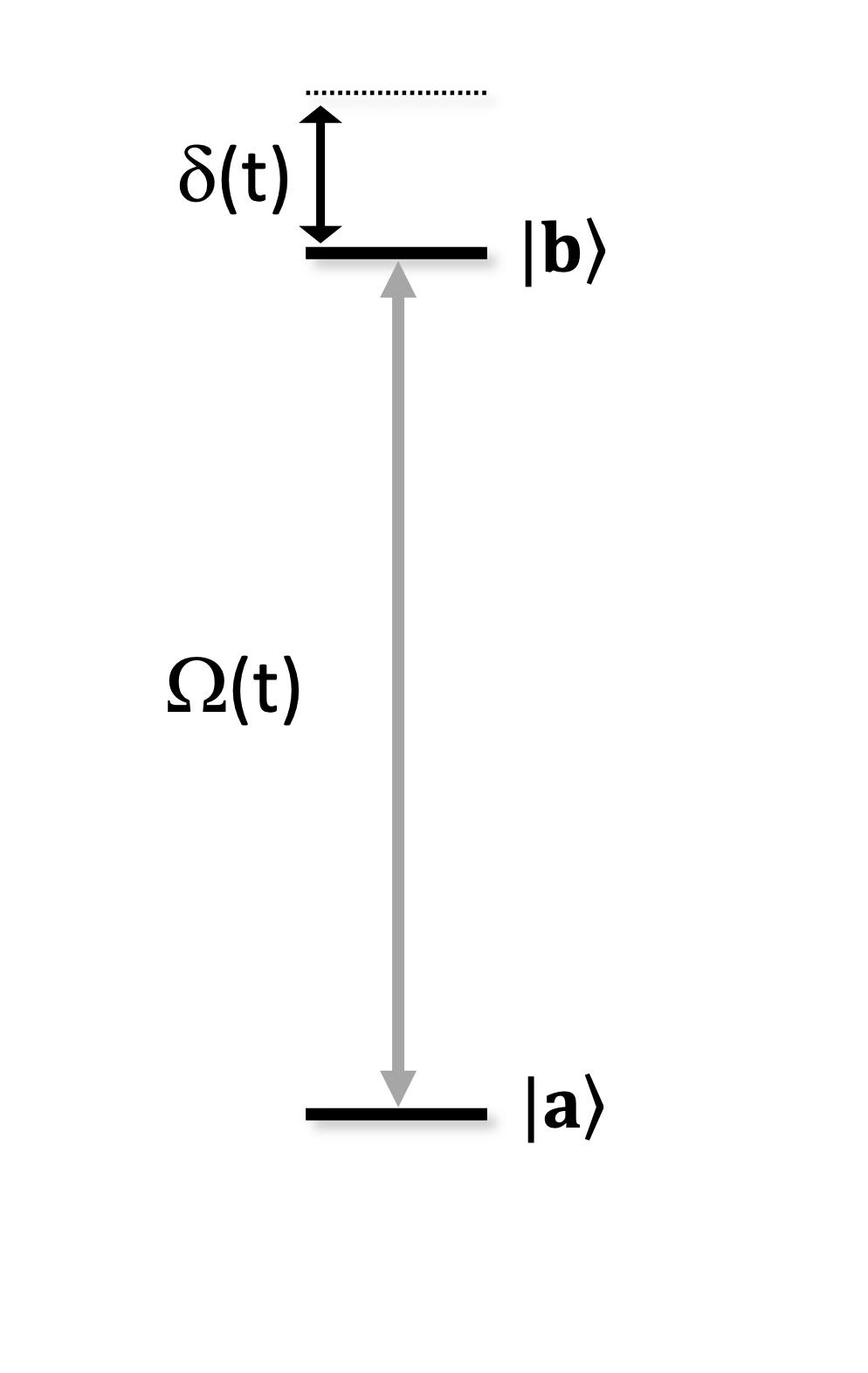# Conventions

## States and Bases

### Bases

A basis refers to a set of two eigenstates. The transition between these two states is said to be addressed by a channel that targets that basis. Namely:

Basis

Eigenstates

Channel type

ground-rydberg

$$|g\rangle,~|r\rangle$$

Rydberg

digital

$$|g\rangle,~|h\rangle$$

Raman

XY

$$|0\rangle,~|1\rangle$$

Microwave

### Qutrit state

The qutrit state combines the basis states of the ground-rydberg and digital bases, which share the same ground state, $$|g\rangle$$. This qutrit state comes into play in the digital approach, where the qubit state is encoded in $$|g\rangle$$ and $$|h\rangle$$ but then the Rydberg state $$|r\rangle$$ is accessed in multi-qubit gates.

The qutrit state’s basis vectors are defined as:

$|r\rangle = (1, 0, 0)^T,~~|g\rangle = (0, 1, 0)^T, ~~|h\rangle = (0, 0, 1)^T.$

### Qubit states

Warning

There is no implicit relationship between a state’s vector representation and its associated measurement value. To see the measurement value of a state for each measurement basis, see State Preparation and Measurement .

When using only the ground-rydberg or digital basis, the qutrit state is not needed and is thus reduced to a qubit state. This reduction is made simply by tracing-out the extra basis state, so we obtain

• ground-rydberg: $$|r\rangle = (1, 0)^T,~~|g\rangle = (0, 1)^T$$

• digital: $$|g\rangle = (1, 0)^T,~~|h\rangle = (0, 1)^T$$

On the other hand, the XY basis uses an independent set of qubit states that are labelled $$|0\rangle$$ and $$|1\rangle$$ and follow the standard convention:

• XY: $$|0\rangle = (1, 0)^T,~~|1\rangle = (0, 1)^T$$

### Multi-partite states

The combined quantum state of multiple atoms respects their order in the Register. For a register with ordered atoms (q0, q1, q2, ..., qn), the full quantum state will be

$|q_0, q_1, q_2, ...\rangle = |q_0\rangle \otimes |q_1\rangle \otimes |q_2\rangle \otimes ... \otimes |q_n\rangle$

Note

The atoms may be labelled arbitrarily without any inherent order, it’s only the order with which they are stored in the Register (as returned by Register.qubit_ids) that matters .

## State Preparation and Measurement

Initial State and Measurement Conventions

Basis

Initial state

Measurement

ground-rydberg

$$|g\rangle$$

$$|r\rangle \rightarrow 1$$
$$|g\rangle,|h\rangle \rightarrow 0$$

digital

$$|g\rangle$$

$$|h\rangle \rightarrow 1$$
$$|g\rangle,|r\rangle \rightarrow 0$$

XY

$$|0\rangle$$

$$|1\rangle \rightarrow 1$$
$$|0\rangle \rightarrow 0$$

### Measurement samples order

Measurement samples are returned as a sequence of 0s and 1s, in the same order as the atoms in the Register and in the multi-partite state.

For example, a four-qutrit state $$|q_0, q_1, q_2, q_3\rangle$$ that’s projected onto $$|g, r, h, r\rangle$$ when measured will record a count to sample

• 0101, if measured in the ground-rydberg basis

• 0010, if measured in the digital basis

## Hamiltonians

Independently of the mode of operation, the Hamiltonian describing the system can be written as

$H(t) = \sum_i \left (H^D_i(t) + \sum_{j<i}H^\text{int}_{ij} \right),$

where $$H^D_i$$ is the driving Hamiltonian for atom $$i$$ and $$H^\text{int}_{ij}$$ is the interaction Hamiltonian between atoms $$i$$ and $$j$$. Note that, if multiple basis are addressed, there will be a corresponding driving Hamiltonian for each transition.

### Driving Hamiltonian

The driving Hamiltonian describes the coherent excitation of an individual atom between two energies levels, $$|a\rangle$$ and $$|b\rangle$$, with Rabi frequency $$\Omega(t)$$, detuning $$\delta(t)$$ and phase $$\phi(t)$$.The coherent excitation is driven between a lower energy level, $$|a\rangle$$, and a higher energy level, $$|b\rangle$$, with Rabi frequency $$\Omega(t)$$ and detuning $$\delta(t)$$.

Warning

In this form, the Hamiltonian is independent of the state vector representation of each basis state, but it still assumes that $$|b\rangle$$ has a higher energy than $$|a\rangle$$.

$H^D(t) / \hbar = \frac{\Omega(t)}{2} e^{-i\phi(t)} |a\rangle\langle b| + \frac{\Omega(t)}{2} e^{i\phi(t)} |b\rangle\langle a| - \delta(t) |b\rangle\langle b|$

#### Pauli matrix form

A more conventional representation of the driving Hamiltonian uses Pauli operators instead of projectors. However, this form now depends on the state vector definition of $$|a\rangle$$ and $$|b\rangle$$.

##### Pulser’s state-vector definition

In Pulser, we consistently define the state vectors according to their relative energy. In this way we have, for any given basis, that

$|b\rangle = (1, 0)^T,~~|a\rangle = (0, 1)^T$

Thus, the Pauli and excited state occupation operators are defined as

$\begin{split}\hat{\sigma}^x = |a\rangle\langle b| + |b\rangle\langle a|, \\ \hat{\sigma}^y = i|a\rangle\langle b| - i|b\rangle\langle a|, \\ \hat{\sigma}^z = |b\rangle\langle b| - |a\rangle\langle a| \\ \hat{n} = |b\rangle\langle b| = (1 + \sigma_z) / 2\end{split}$

and the driving Hamiltonian takes the form

$H^D(t) / \hbar = \frac{\Omega(t)}{2} \cos\phi(t) \hat{\sigma}^x - \frac{\Omega(t)}{2} \sin\phi(t) \hat{\sigma}^y - \delta(t) \hat{n}$
##### Alternative state-vector definition

Outside of Pulser, the alternative definition for the basis state vectors might be taken:

$|a\rangle = (1, 0)^T,~~|b\rangle = (0, 1)^T$

This changes the operators and Hamiltonian definitions, as rewriten below with highlighted differences.

$\begin{split}\hat{\sigma}^x = |a\rangle\langle b| + |b\rangle\langle a|, \\ \hat{\sigma}^y = \textcolor{red}{-}i|a\rangle\langle b| \textcolor{red}{+}i|b\rangle\langle a|, \\ \hat{\sigma}^z = \textcolor{red}{-}|b\rangle\langle b| \textcolor{red}{+} |a\rangle\langle a| \\ \hat{n} = |b\rangle\langle b| = (1 \textcolor{red}{-} \sigma_z) / 2\end{split}$
$H^D(t) / \hbar = \frac{\Omega(t)}{2} \cos\phi(t) \hat{\sigma}^x \textcolor{red}{+}\frac{\Omega(t)}{2} \sin\phi(t) \hat{\sigma}^y - \delta(t) \hat{n}$

Note

A common case for the use of this alternative definition arises when trying to reconcile the basis states of the ground-rydberg basis (where $$|r\rangle$$ is the higher energy level) with the computational-basis state-vector convention, thus ending up with

$|0\rangle = |g\rangle = |a\rangle = (1, 0)^T,~~|1\rangle = |r\rangle = |b\rangle = (0, 1)^T$

### Interaction Hamiltonian

The interaction Hamiltonian depends on the states involved in the sequence. When working with the ground-rydberg and digital bases, atoms interact when they are in the Rydberg state $$|r\rangle$$:

$H^\text{int}_{ij} = \frac{C_6}{R_{ij}^6} \hat{n}_i \hat{n}_j$

where $$\hat{n}_i = |r\rangle\langle r|_i$$ (the projector of atom $$i$$ onto the Rydberg state), $$R_{ij}^6$$ is the distance between atoms $$i$$ and $$j$$ and $$C_6$$ is a coefficient depending on the specific Rydberg level of $$|r\rangle$$.

On the other hand, with the two Rydberg states of the XY basis, the interaction Hamiltonian takes the form

$H^\text{int}_{ij} = \frac{C_3}{R_{ij}^3} (\hat{\sigma}_i^{+}\hat{\sigma}_j^{-} + \hat{\sigma}_i^{-}\hat{\sigma}_j^{+})$

where $$C_3$$ is a coefficient that depends on the chosen Ryberg states and

$\hat{\sigma}_i^{+} = |1\rangle\langle 0|_i,~~~\hat{\sigma}_i^{-} = |0\rangle\langle 1|_i$

Note

The definitions given for both interaction Hamiltonians are independent of the chosen state vector convention.